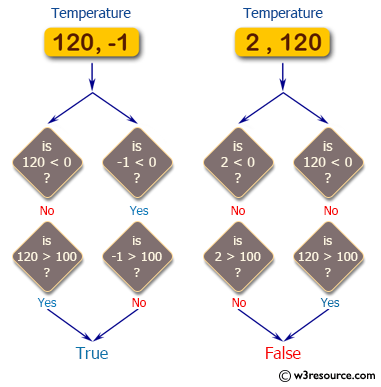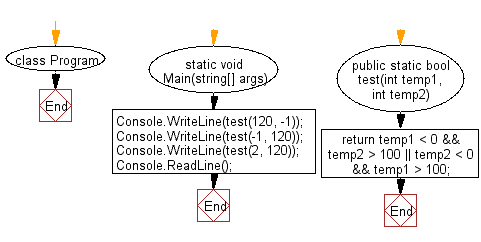﻿ C# - Check temperatures below 0 and above 100# C# Sharp Basic Algorithm Exercises: Check whether one of the given temperatures is less than 0 and the other is greater than 100

## C# Sharp Basic Algorithm: Exercise-13 with Solution

Write a C# Sharp program to check whether one of the given temperatures is less than 0 and the other is greater than 100.

Pictorial Presentation:Sample Solution:

C# Sharp Code:

``````using System;

namespace exercises
{
class Program
{
static void Main(string[] args)
{
Console.WriteLine(test(120, -1));
Console.WriteLine(test(-1, 120));
Console.WriteLine(test(2, 120));
}

public static bool test(int temp1, int temp2)
{
return temp1 < 0 && temp2 > 100 || temp2 < 0 && temp1 > 100;
}
}
}
```
```

Sample Output:

```True
True
False```

Flowchart:C# Sharp Code Editor:

Improve this sample solution and post your code through Disqus

What is the difficulty level of this exercise?

Test your Programming skills with w3resource's quiz.

﻿